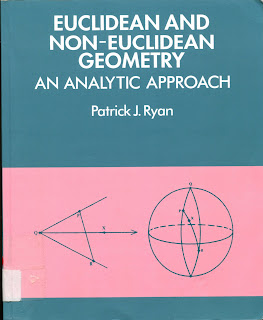GEOMETRY MATHEMATICS

# Euclidean and Non Euclidean Geometry An Analytic Approach PDF Free DownloadThis book gives a rigorous treatment of the fundamentals of plane geometry: Euclidean, spherical, elliptical and hyperbolic. The primary purpose is to acquaint the reader with the classical results of plane Euclidean and non Euclidean geometry, congruence theorems, concurrence theorems, classification of isometries, angle addition and trigonometrical formulae. However, the book not only provides students with facts about and an understanding of the structure of the classical geometries, but also with an arsenal of computational techniques for geometrical investigations. The aim is to link classical and modern geometry to prepare students for further study and research in group theory, Lie groups, differential geometry, topology, and mathematical physics. The book is intended primarily for undergraduate mathematics students who have acquired the ability to formulate mathematical propositions precisely and to construct and understand mathematical arguments. Some familiarity with linear algebra and basic mathematical functions is assumed, though all the necessary background material is included in the appendices.